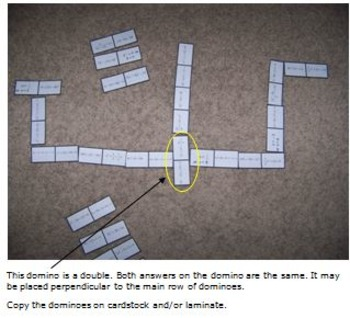# MATH DOMINOES - Solve Quadratic Equations8th - 11th
Subjects
Resource Type
Formats Included
• PDF
Pages
N/A

### Description

This game is a fun way for your students to practice solving quadratic equations. There are 21 dominoes with either a problem on both ends or a problem and a solution to a quadratic equation.

There are six different solutions to all the questions, so just like regular dominoes there are many ways the dominoes may be played. There are 36 different quadratic equations presented on the dominoes. All of the equations can be factored; however, students may solve the equations using any strategy they prefer. Please view the difficulty level of the equations in the preview.

See the preview for instructions to play the game.

The solution of each equation is provided on a separate set of dominoes so that you can quickly check that students are matching dominoes correctly.

Click on a title below to view additional math resources.

MATH DOMINOES - Solve Linear Equations

Math Board Game - Pythagorean Theorem

High School Algebra Board Game - Linear and Quadratic Equations

Math Board Game - Trigonometry - Trigonometric Ratios and Solve Right Triangles

Math Functions Lesson - High School Algebra

Thanks for viewing!

Created by Hilda Ratliff
Total Pages
N/A
Included
Teaching Duration
N/A
Report this Resource to TpT
Reported resources will be reviewed by our team. Report this resource to let us know if this resource violates TpT’s content guidelines.